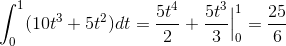# Calculus 3 : Line Integrals of Vector Fields

## Example Questions

### Example Question #1 : Line Integrals Of Vector Fields

Evaluate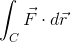, where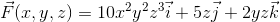, andis the curve given by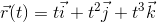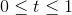.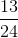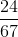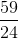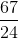Explanation:

First we need to evaluate the vector field evaluated along the curve.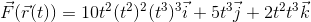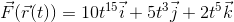Now we need to find the derivative of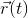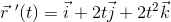Now we can do the product of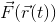and.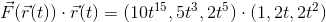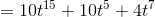Now we can put this into the integral and evaluate it.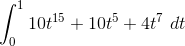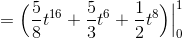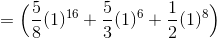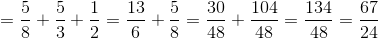### Example Question #2 : Line Integrals Of Vector Fields

Find the work done by a particle moving in a force field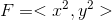, moving from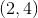to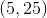on the path given by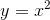.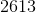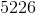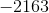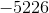Explanation:

The formula for work is given by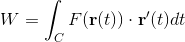.

Writing our path in parametric equation form, we have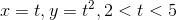.

Hence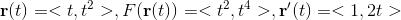Plugging this into our work equation, we get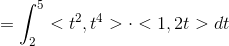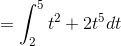.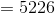### Example Question #3 : Line Integrals Of Vector Fields

Evaluate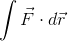on the curve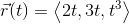, where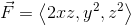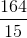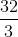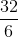Explanation:

The line integral of a vector field is given by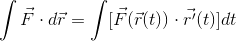So, we must evaluate the vector field on the curve: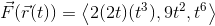Then, we take the derivative of the curve with respect to t: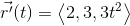Taking the dot product of these two vectors, we get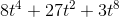This is the integrand of our integral. Integrating, we get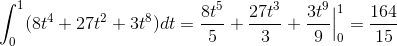### Example Question #4 : Line Integrals Of Vector Fields

Evaluate the integralon the curve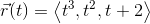, where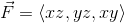, on the interval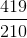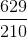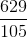Explanation:

The line integral of the vector field is equal to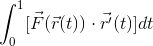The parameterization (using the corresponding elements of the curve) of the vector field is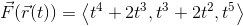The derivative of the parametric curve is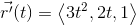Taking the dot product of the two vectors, we get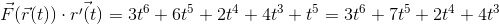Integrating this with respect to t on the given interval, we get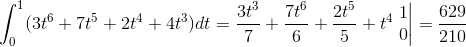### Example Question #5 : Line Integrals Of Vector Fields

Calculateon the interval, where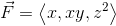and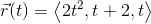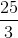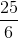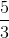Explanation:

To calculate the line integral of the vector field, we must evaluate the vector field on the curve, take the derivative of the curve, and integrate the dot product on the given interval.

The vector field evaluated on the given curve is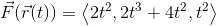The derivative of the curve is given by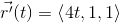The dot product of these is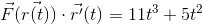Integrating this over our given t interval, we get